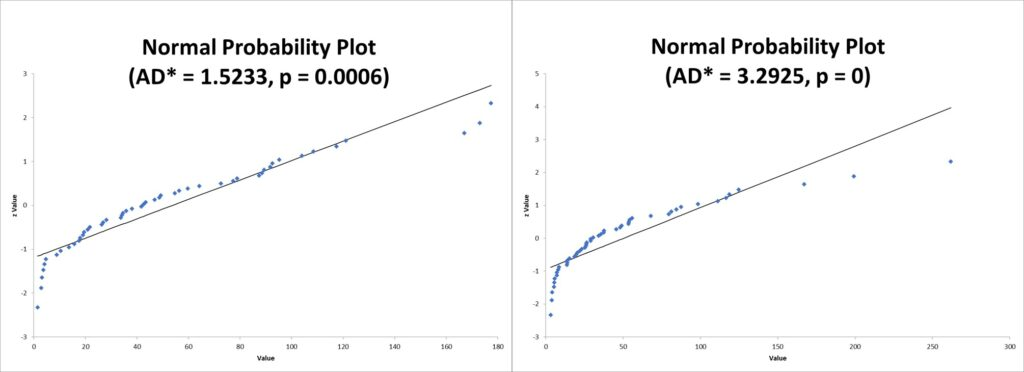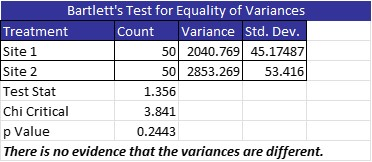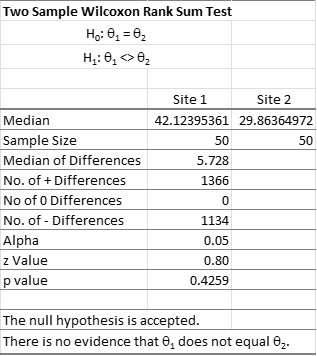The Wilcoxon Rank Sum Test, sometimes called the Mann Whitney Wilcoxon Test or Mann Whitney U test, is used to test whether two independent samples come from the same population or two different populations.

Since the Wilcoxon Rank Sum Test is a form of hypothesis testing, there will be an associated null and alternative hypothesis. The test is used for continuous data. Let’s learn a little more about the Wilcoxon Rank Sum Test.

## Overview: What is the Wilcoxon Rank Sum Test?

The test is named for Frank Wilcoxon (1892–1965) who was a chemist and statistician. The Wilcoxon Rank Sum Test is often described as the non-parametric version of the 2-sample t-test. Recall that the 2-sample t test is used for comparing two population means. The underlying assumptions of the 2-sample t-test are that:

1. The two samples are independent of each other
2. The two populations have equal variance
3. The two populations are normally distributed

The first assumption must be satisfied for a 2-sample t-test. When the assumptions of equal variance and normality are not satisfied, but the samples are large, you can say the assumptions are robust and your results are approximately correct. But when your samples are small and your data is skewed or non-normal, you should consider a Plan B..

The Wilcoxon Rank Sum Test is your Plan B. It only requires the first two assumptions of independence and equal variance. It does not assume your data has a known distribution. Since the Wilcoxon Rank Sum Test does not assume a known distribution, it does not deal with parameters, and therefore it is called a non-parametric test.

Whereas the null hypothesis of the 2-sample t-test is equal population means, the null hypothesis of the Wilcoxon tests for equal population medians. If you reject the null, that means you have evidence that the two population medians from where the samples were taken are different. The Wilcoxon test, and its variations, can be used as a 1-sample, 2-sample (independent) or 2-sample (paired) test.

## An industry example of the Wilcoxon Rank Sum Test

The production manager was trying to compare the performance of two sites. He randomly collected two sets of independent data. His Master Black Belt suggested he use a 2-sample t-test if the assumptions are met. Unfortunately, as you can see below, neither of the data sets were normally distributed.It was then suggested he try using a Wilcoxon Rank Sum Test. The assumption of equal variances was tested, and the graphic below shows the two groups of data have equal variances.Now that the assumptions have been fulfilled, the manager ran the Wilcoxon Rank Sum Test. As you can see, there was no difference between the two sites.

##Frequently Asked Questions (FAQ) about the Wilcoxon Rank Sum Test

### What are the two assumptions of the Wilcoxon Rank Sum Test for two groups of data?

The first assumption is that the two groups of sample data are independent. The second assumption is that the two groups have equal variances. Since this test is a non-parametric test, there is no assumption regarding a distribution.

### What is the Wilcoxon Rank Sum Test used for?

This test is used to determine whether the medians of the populations from which sample data was selected are equal or not.

### What is the difference between the Wilcoxon Signed Rank Test and the Wilcoxon Rank Sum Test?

The Signed Rank test compares medians of a paired set of data. The Rank Sum test is used for two independent sample groups.